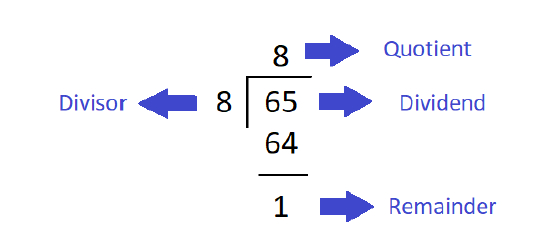# Java Program to Compute Quotient and Remainder

JavaObject Oriented ProgrammingProgramming

In this article, we will understand how to compute the quotient and reminder in Java. Quotient and Reminder is calculated using two simple formula "Quotient = Dividend / Divisor" and "Remainder = Dividend % Divisor".

Given an integer a and a non-zero integer d, it can be shown that there exist unique integers q and r, such that a = qd + r and 0 ≤ r < |d|. The number q is called the quotient, while r is called the remainder.

Below is a demonstration of the same −Input

Suppose our input is −

Dividend value: 50
Divisor: 3

Output

The desired output would be −

Quotient: 16
Remainder: 2

## Algorithm

Step1- Start
Step 2- Declare four integers as my_dividend , my_divisor, my_quotient, my_remainder
Step 3- Prompt the user to enter two integer value that is my_dividend , my_divisor or define
the integers
Step 5- Use the formula to find the quotient and the reminder "Quotient = Dividend /
Divisor" and "Remainder = Dividend % Divisor"
Step 6- Display the result
Step 7- Stop

## Example 1

Here, the input is being entered by the user based on a prompt. You can try this example live in ourcoding ground tool.

import java.util.Scanner;
public class RemainderQuotient {
public static void main(String[] args) {
int my_dividend , my_divisor, my_quotient, my_remainder;
System.out.println("Required packages have been imported");
Scanner my_scanner = new Scanner(System.in);
System.out.println("A scanner object has been defined ");
System.out.print("Enter the value of dividend : ");
my_dividend = my_scanner.nextInt();
System.out.print("Enter the value of divisor : ");
my_divisor = my_scanner.nextInt();
my_quotient = my_dividend / my_divisor;
my_remainder = my_dividend % my_divisor;
System.out.println("The quotient is " + my_quotient);
System.out.println("The remainder is " + my_remainder);
}
}

## Output

Required packages have been imported
A reader object has been defined
Enter the value of dividend : 50
Enter the value of divisor : 3
The quotient is 16
The remainder is 2

## Example 2

Here, the integer has been previously defined, and its value is accessed and displayed on the console.

public class RemainderQuotient {
public static void main(String[] args) {
int my_dividend , my_divisor, my_quotient, my_remainder;
my_dividend = 50;
my_divisor = 3;
System.out.println("The divident and the divisor are defined as " +my_dividend +" and " +my_divisor);
my_quotient = my_dividend / my_divisor;
my_remainder = my_dividend % my_divisor;
System.out.println("The quotient is " + my_quotient);
System.out.println("The remainder is " + my_remainder);
}
}

## Output

The divident and the divisor are defined as 50 and 3
The quotient is 16
The remainder is 2